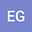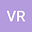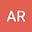Influence of the Rosenzweig functional response on the dynamics of the Leslie-Gower model
•••• +1
• EDUARDO GONZALEZ-OLIVARES,
• Viviana Rivera-Estay,
• Alejandro Rojas,
• Karina VILCHES-PONCE
EDUARDO GONZALEZ-OLIVARES

Corresponding Author:eduardo.gonzalez@pucv.cl

Author ProfileViviana Rivera-Estay
Author ProfileAlejandro RojasAfter the well-known classification formulated by Crawford S. Holling in 1959 of the functional responses dependent only of the prey populations, various other have been proposed. In this work a simple Leslie-Gower type predator-prey model is analyzed, incorporating the Rosenzweig functional response described by $h\left( x\right) =qx^{\alpha }$, with $0<\alpha <1$. This function does not conform to the types proposed by Holling, since is not bounded. Although this functional response is non-differentiable for $x=0$, it is proved that the obtained system is Lipschitzian. However, the existence of a separatrix curve $\Sigma$ in the phase plane it is proven, which divides the phase plane en two complemntary sectors. According to the relative position of the initial conditions respect to the curve $\Sigma$ , the trajectories can have differents $\omega$-$limit$, which can be the equilibrium $\left( 0,0\right)$, or else, a positive equilibrium point, or a limit cycle or a heteroclinic curve. These properties show the great diffference of this model with the original and well-known Leslie-Gower model (when $\alpha =1$), since this last has only a unique positive equilibrium, which is globally asymptotically stable. Then, it can concluded that i) a small change in the mathematical expression for the functional response, it produces a strong change on the dynamics of model. ii) \ a slightest deviation in the initial population sizes, respect to the curve $\Sigma$, it can signify the coexistence of populations or the extinction of both. Numerical simulations are given to endorse our analytical results.